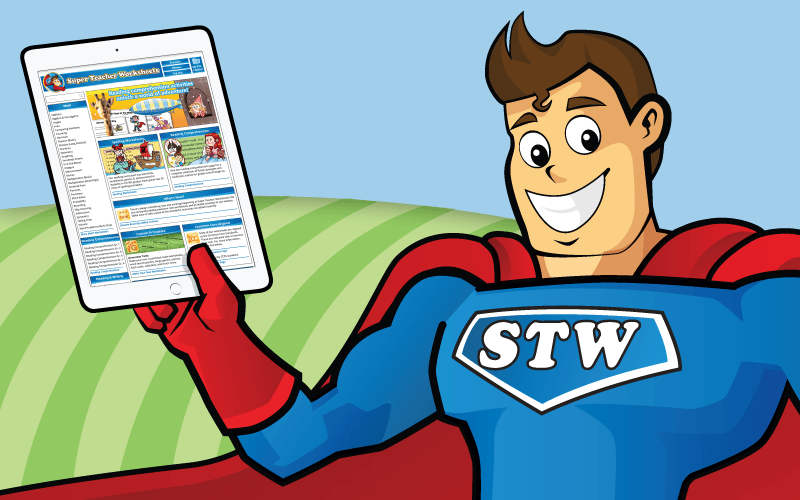# Decimal Place Value: Hundredths

The worksheets and activities on this page can be used for teaching students about decimal place value to the nearest hundredth place.## Decimals to Hundredths

The numbers in this worksheet go to tenths and hundredths. In the first part, students will determine the value of the underlined digit. In the second part, they will answer questions related to the place values in a particular number.
Tell which word name corresponds with the standard form decimal number.
Students will practice place value by following instructions to color the numbers in each place a certain color. Then they will cut out the word boxes and place them over the corresponding digits in the number.

Students must correctly read aloud the decimal amounts. Includes numbers with both tenths and hundredths.
Print this file on card stock. Attach the two parts of the wheel with a paper fastener. Spin the wheel and read the decimal numbers. (example: 4.12 is "four and twelve hundredths")
Here is another number wheel to help students practice reading decimal numbers aloud.

## Comparing Decimals

Use the symbols greater than, less than, and equal to symbols to compare the decimal amounts.  Includes decimals in tenths and hundredths.  (example:  9.8 > 4.5)
Use greater than, less than, and equal to symbols to show which decimal is bigger.  Decimals are in tenths and hundredths.  (example: 1.01 < 1.11)

## Ordering Decimals

This worksheet has whole numbers, decimals to the nearest tenth, and decimals to the nearest hundredth. Students determine their values and list them from least to greatest.
Arrange the decimal number cards in order from smallest value to greatest value. This worksheet has whole numbers, as well as decimals in tenths and hundredths.
More Place Value Worksheets

Super Teacher has place value activities for all levels. We have pages for all numbers of digits, from 2 through 10, as well as decimal and money.

My Account
Site Information